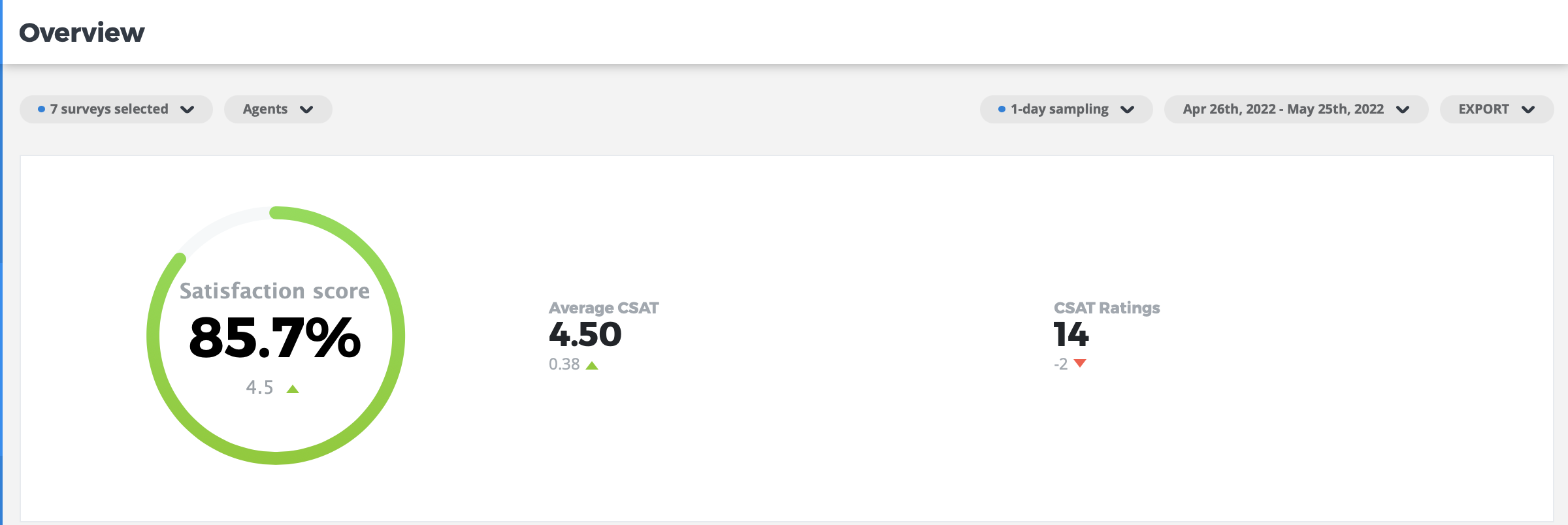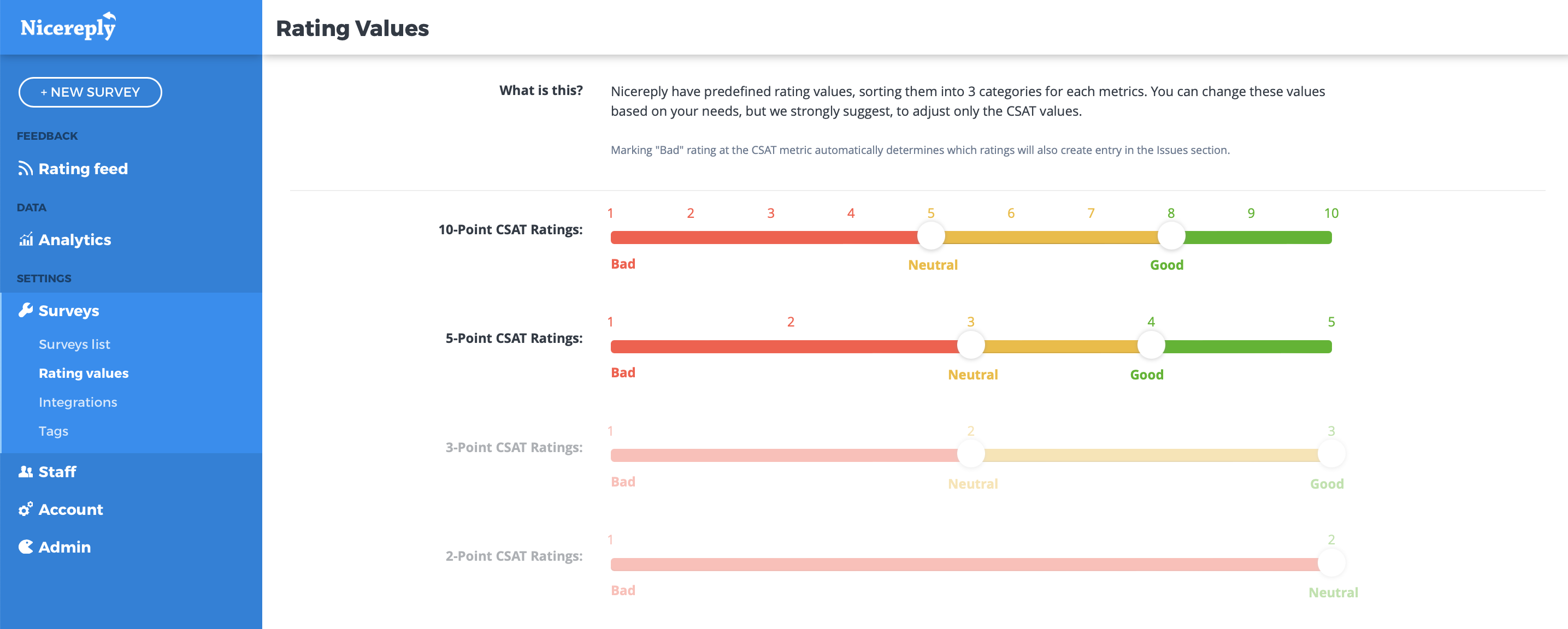# How is CSAT calculated?

To calculate the CSAT score, subtract the % of customers who were unhappy from 100%.

We calculate the CSAT score by the standard formula.

The formula is  (The total number of positive responses) ÷ (Number of total responses) x 100 = % of satisfied customers

To see this value go to your Nicereply account > Analytics > Overview. The value is called the Satisfaction score

There is also the Average CSAT value which displays the average CSAT rating for a selected period.You can define the positive responses (what is a good rating and what is a bad rating) when you go to SURVEYS  > RATING VALUES. For instance, we consider the score of 4 and 5 as good on the 5-point scale.